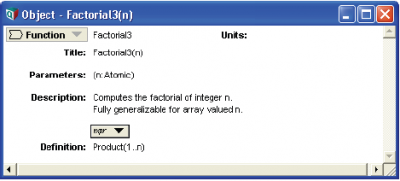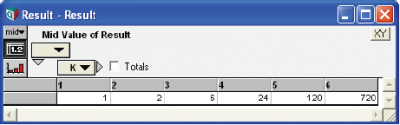# Ensuring Array Abstraction

The vast majority of the elements of the Analytica language (operators, functions, and control constructs) fully support Intelligent Arrays — that is, they can handle operands or parameters that are arrays with any number of indexes, and generate a result with the appropriate dimensions. Thus, most models automatically obtain the benefits of array abstraction with no special care.

There are just a few elements that do not inherently enable Intelligent Arrays — i.e., support array abstraction. They fall into these main types:

When using these constructs, you must take special care to ensure that your model is fully arrayabstractable. Here we explain how to do this for each of these five types.

## Functions Expecting Atomic Parameters

Consider this example:

Variable N := 1..3
Variable B := 1..N
B →
Evaluation error: One or both parameters to Sequence(m, n) or m..n are not scalars.

The expression 1..N, or equivalently, Sequence(1, N), cannot work if N is an array, because it would have to create a nonrectangular array containing slices with 1, 2, and 3 elements. Analytica does not allow nonrectangular arrays, and so requires the parameters of Sequence to be atoms (single elements).

Most functions and expressions that, like Sequence, are used to generate the definition of an index require atomic (or in some cases, vector) parameters, and so are not fully array abstractable. These include Sequence, Subset, SplitText, SortIndex (if the second parameter is omitted), Concat, CopyIndex, and Unique.

Why would you want array abstraction using such a function? Consider this approach to writing a function to compute a factorial:

Function Factorial2
Parameters: (n)
Definition: Product(1..n)

It works if n is an atom, but not if it is an array, because 1..n requires atom operands. In this version, however, using a For loop works fine:

Function Factorial3
Parameters: (n)
Definition: FOR m := n DO Product(1..m)

The For loop repeats with the loop variable m set to each atom of n, and evaluates the body Product(1..m) for each value. Because m is guaranteed to be an atom, this works fine. The For loop reassembles the result of each evaluation of Product(1..m) to create an array with all the same dimensions as n.

## Atom parameters and array abstraction

Another way to ensure array abstraction in a function is to use the Atom qualifier for its parameter(s). When you qualify a parameter n as an Atom, you are saying that it must be a single value — not an array — when the function is evaluated, but not when the function is used:

Function Factorial3
Parameters: (n: Atom)
Definition: Product(1..n)Index K := 1..6
Factorial3(K) →Notice that Atom does not require the actual parameter K to be an atom when the function is called. If K is an array, as in this case, it repeatedly evaluates the function Factorial3(n) with n set to each atom of array K. It then reassembles the results back into an array with the same indexes as parameter K, like the For loop above. This scheme works fine even if you qualify several parameters of the function as Atom.

In some cases, a function might require a parameter to be an vector (have only one index), or have multiple dimensions with specified indexes. You can use Array qualifiers to specify this. With this approach, you can ensure your function array abstracts when new dimensions are added to your model, or if parameters are probabilistic.

## While and array abstraction

The While b Do e construct requires its termination condition «b» to evaluate to be an atom — that is, a single Boolean value, True (1) or False (0). Otherwise, it would be ambiguous about whether to continue. Again, Atom is useful to ensure that a function using a While loop array abstracts, as it was for the Sequence function. Here’s a way to write a Factorial function using a While loop:

Function Factorial4
Parameters: (n: Atom)
Definition:
Local fact := 1, a := 1;
While a < n Do (a := a + 1; fact := fact*a)

In this example, the Atom qualifier assures that n and hence the While termination condition a < n is an atom during each evaluation of Factorial4.

## If a Then b Else c and array abstraction

Consider this example:

Variable X := -2..2
Sqrt(X) → [NAN, NAN, 0, 1, 1.414]

The square root of negative numbers -2 and -1 returns NAN (not a number) after issuing a warning. Now consider the definition of Y:

Variable Y := (If X > 0 Then Sqrt(X) Else 0)
Y → [0, 0, 0, 1 1.414]

For the construct IF a THEN b ELSE c, «a» is an array of truth values, as in this case, so it evaluates both «b» and «c». It returns the corresponding elements of «b» or «c», according to the value of condition «a» for each index value. Thus, it still ends up evaluating Sqrt(X) even for negative values of X. In this case, it returns 0 for those values, rather than NAN, and so it does not generate an error message.

A similar problem remains with text processing functions that require a parameter to be a text value. Consider this array:

Variable Z := [1000, '10,000', '100,000']

This kind of array containing true numbers, e.g., 1000, and numbers with commas turned into text values, often arises when copying arrays of numbers from spreadsheets. The following function would seem helpful to remove the commas and convert the text values into numbers:

Function RemoveCommas(t)
Parameters: (t)
Definition: Evaluate(TextReplace(t, ',', ))
RemoveCommas(Z) →
Evaluation Error: The parameter of Pluginfunction TextReplace must be a text while evaluating function RemoveCommas.

TextReplace doesn’t like the first value of z, which is a number, where it’s expecting a text value. What if we test if t is text and only applies TextReplace when it is?

Function RemoveCommas(t)
Parameters: (t)
Definition: If IsText(t) 
Then Evaluate(TextReplace(t, ',', )) Else t
RemoveCommas(Z) → (same error message)

It still doesn’t work because the If construct still applies TextReplace to all elements of t. Now, let’s add the parameter qualifier Atom to t:

Function RemoveCommas(t)
Parameters: (t: Atom)
Definition: If IsText(t)
Then Evaluate(TextReplace(t, ',', )) Else t
RemoveCommas(Z) →This works fine because the Atom qualifier means that RemoveCommas breaks its parameter t down into atomic elements before evaluating the function. During each evaluation of Remove-Commas, t, and hence IsText(t), is atomic, either True or False. When False, the If construct evaluates the Else part but not the Then part, and so calls TextReplace when t is truly a text value. After calling TextReplace separately for each element, it reassembles the results into the array shown above with the same index as Z.

## Omitted index parameters and array abstraction

Several functions have index parameters that are optional, including Sum, Product, Max, Min, Average, Argmax, SubIndex, ChanceDist, CumDist and ProbDist. For example, with Sum(x, i), you can omit index «i», and call it as Sum(x). But, if «x» has more than one index, it is hard to predict which index it sums over. Even if «x» has only one dimension now, you might add other dimensions later, for example for parametric analysis. This ambiguity makes the use of functions with omitted index parameters non-array abstractable.

There is a simple way to avoid this problem and maintain reliable array abstraction: When using functions with optional index parameters, never omit the index! Almost always, you know what you want to sum over, so mention it explicitly. If you add dimensions later, you’ll be glad you did.

Tip
When the optional index parameter is omitted, and the parameter has more than one dimension, these functions choose the outer index, by default. Usually, the outer index is the index created most recently when the model was built. But, this is often not obvious. We designed Intelligent Arrays specifically to shield you from having to worry about this detail of the internal representation.

## Selecting indexes for iterating with For and Local

To provide detailed control over array abstraction, the For loop can specify exactly which indexes to use in the iterator «x». The old edition of For still works. It requires that the expression «a» assigned to iterator «x» generate an index — that is, it must be a defined index variable, Sequence(m, n), or m..n. The new forms of For are more flexible. They work for any array (or even atomic) value «a». The loop iterates by assigning to «x» successive subarrays of «a», dimensioned by the indexes listed in square brackets. If the square brackets are empty, as in the second line of the table, the successive values of iterator «x» are atoms. In the other cases, the indexes mentioned specify the dimensions of «x» to be used in each evaluation of «e». In all cases, the final result of executing the For loop is a value with the same dimensions as «a».

Construct Meaning
For x := a Do e Assigns to loop variable «x» successive atoms from index expression «a» and repeats evaluation expression «e» for each value. Returns an array of values of «e» indexed by «a».
For x := a Do e

For x[] := a Do e

Assigns to loop variable «x», successive atomic values from array «a». It repeats evaluation of expression «e» for each value. It returns an array of values of «e» with the same indexes as «a».
For x[i] := a Do e Assigns to loop variable «x» successive subarrays from array «a», each indexed only by «i». It repeats evaluation of expression «e» for each index value of «a» other than «i». As before, the result has the same indexes as «a».
For x[i, j …] := a Do e Assigns to loop variable «x» successive subarrays from array «a», each indexed only by «i», «j».... It repeats evaluation of expression «e» for each index value of «a» other than «i», «j» …. As before, the result has the same indexes as «a».

The same approach also works using Var to define local variables. By putting square brackets listing indexes after the new variable, you can specify the exact dimensions of the variable. These indexes should be a subset (none, one, some, or all) of the indexes of the assigned value «a». Any subsequent expressions in the context are automatically repeated as each subarray is assigned to the local variable. In this way, a local variable can act as an implicit iterator, like the For loop.

Local Temp[i1, i2, ...] := X;